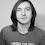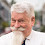## Wednesday, November 23, 2016

### Music Theory for Theorists

I fully completed and passed my first MOOC, Fundamentals of Music Theory, a Coursera course out of the University of Edinburgh. I played the tuba in college and grad school but really just practiced scales and (usually) hit the notes. I didn't really understand keys, intervals, chords and the such and I wanted to learn.

Music theory has the same problem as C++, too much overloaded notation before you really understand what's going on. So here's a quick view of music theory without the mess.

Think (theoretically) of an bi-infinite sequence of notes. Every note is equivalent to notes a factor of twelve away from them (though in different octaves). Pick a note, label it 1, then the notes labelled 1, 3, 5, 6, 8, 10, 12, 1 (an octave higher) form a major scale in the key of note 1. There are twelve major scales, depending on which note you label 1. If you start at 10, i.e., 10, 12, 1, 3, 5, 6, 8, 10 you get the relative minor scale in the key of note 10. Also twelve minor scales.

Start a new major scale starting from 8 you'll get 8, 10, 12, 1, 3, 5, 7, 8, just one number off from the previous scale. Repeating this process will run through all the major scales. This is called the circle of fifths (since 8 is the fifth note of the scale). You can go through the circle backwards by starting at 6.

A chord is typically three or four alternating notes of a scale, for example 1, 5, 8 (the tonic) and 8, 12, 3, 6 (the dominant 7th). They can be inverted using different octaves and all sorts of other good stuff.

So notation wise, there is a mapping of {A,B,C,D,E,F,G} X {Flat, Natural, Sharp} to the twelve notes with several notes getting two different names in such a way that each note of any scale can get one of the letters in cyclic order. For example the D-Major scale would be the notes D-Natural, E-Natural, F-Sharp, G-Natural, A-Natural, B-Natural, C-Sharp and back to D-Natural. You put it all together in a diagram called a musical score.

Many undergrad computing courses now use Python instead of C++ to get around the notational challenges. Alas music notation goes back a millennium or so, I don't expect any changes soon.

1.One thing which is still confusing in your notation is that since "every note is equivalent to notes a factor of twelve away from them" one would expect 12 and 1 to have the same name (since 12=12*1).

It could be better to use an additive notation, so that the notes would be 0,1,...,11,12=0,...

2.Historically the overloaded system had meaning - C-sharp or D-flat weren't exactly the same, because the notes "veered" a bit according to their place in the scale from the current "Well-tempered" tuning system.

I'm not 100% sure, I think the original Pythagorean system produced frequencies proportional to 3^k2^l (positive and negative k,l, the subset depending on the scale), while now things are "rounded" to 2^(k/12) (all integer k).

3.For fretted instruments (especially in Flamenco guitar), the Tablature notation is more natural/popular: https://en.wikipedia.org/wiki/Tablature

4.You will need to introduce sharps and flats when you start comparing scales. E.g. If you compare C major and F major you'll se one note flatted in F major (B). This is also a way to introduce scale of fifth: sequence of scales with difference 1. If you adopt this differential look then sharps and flats start to look a little more reasonable.

5.A useful fact, especially for understanding key signatures, is that a major scale consists of an arithmetic progression with common difference 7 and length 7 (mod 12). Of course, it's not played in that order.

6.Following Eldar Fischer: scales provide a family of notes that, in some sense, sound good together, as determined by the ratio of their frequencies: ratios of two mean the notes are so "close" that they might as well be the same. Since we hear these ratios logarithmically, a given ratio P is heard as
(log_2 P) mod 1.
Ratios that are powers of 3 are also nice, so we like families of notes with frequency ratios of the form 3^k for integer k. We hear these as
(k*R) mod 1, where R=log_2(3).

Since R = 19.02/12 to that many digits precision, we have 12R very close to zero mod 1. That is, since 3/2 is very close to 2^(7/12), if we have a family of notes of the form 2^(k/12), for any given k the note 7 steps up has a ratio 2^(7/12) = 3/2 - 0.0017, the interval of a "fifth". Similarly the note 5 steps, up a "fourth", has 2^(5/12) = 4/3 + 0.002. Also the note 4 steps up, a "third", has the ratio 2^(4/12) = 5/4 + 0.06, not related to log_2(3) but still a small integer multiple of 2^i.

So steps 4,5, and 7 give pretty good small integer ratios of frequencies, up to powers of two. If you throw in 11, a fifth above the third or a third above the fifth, and 9, a third above the fourth and vice versa, and 2, a fourth below the fifth and vice versa, you get 7 notes with many "nice" subsets. With k=12, that makes an octave or "scale".

For any k mod 12, there's a similar family of 8. Visiting such families by looking at them in ratios of 3/2, that is, 7*k mod 12, gives the "circle of "fifths".

But the main thing, I think, is that 12*log_2(3) is close to an integer. As I noted in an earlier discussion at Bernard Chazelle's blog, up to bugs in my little python program, k=12 is among the best 5% of integers less than 1000 with respect to this difference, and among those less than 100, only 53, 94, and 41 are better. Among the k less than 12, the next best is k=5, and the distance of 5 log_2(3) to an integer is four times larger than the distance for k=12.

1.Your comment wasn't visible when I posted mine. For k < 12, k=7 is closer than k=5. There are integer relations here, e.g. 5 + 7 = 12, which can be visualized with the Stern-Brocot tree. There is also a relationship with the zeta function

http://oeis.org/A117536
http://oeis.org/A117538
http://xenharmonic.wikispaces.com/The+Riemann+Zeta+Function+and+Tuning

The tuning of 5 is also important in triads, 7 in barbershop quartet singing, and 11 and higher primes in the music of Harry Partch, Ben Johnston, and others.

The tuning of 2 can also be an approximation, so k does not have to be an integer.

When there are 3 or more primes, an approximation is not fully determined by k. We need a vector, which can be written as a bra vector, called a "val" in the parlance.

But some historical tuning systems like the "meantone" temperament can't be described by any vector. Two vectors are required, making them "rank 2 regular temperaments" in the parlance.

Various ways of comparing these systems have been explored in some depth...

7.The interested person may like recent work generalizing music theory beyond 12-tone equal temperament

http://xenharmonic.wikispaces.com/Mathematical+Theory

I'll subscribe to replies here and try to answer any questions.

8.1. Chords (and their inversions) in themselves are unimportant and uninteresting. What matters is their use (especially in the common era) as steps in a harmonic structure.
2. Tuning is much more than the mathematical ways described by carl. Pythagorean tuning produces unevenness that is clearly audible, and makes transposed versions sound pretty weird. The answer was not necessarily dividing the octave into 12 equal parts, but many families of "temperaments" (hacked fudge factors) that changed the equal temperament in ways that were more or less logical and more or less pleasant. Music history is full of very heated discussions, by theorist from the 16th to the 20th centuries arguing about the best ways to do this. Many of the arguments were not about Physics, but musical sentiment, expressiveness, etc. The Grove Dictionary (on-line, but with paywall) will tell you more than you ever wanted to know....

9.Why 12 notes? Because (3/2)^12 ~= 2^7

10.